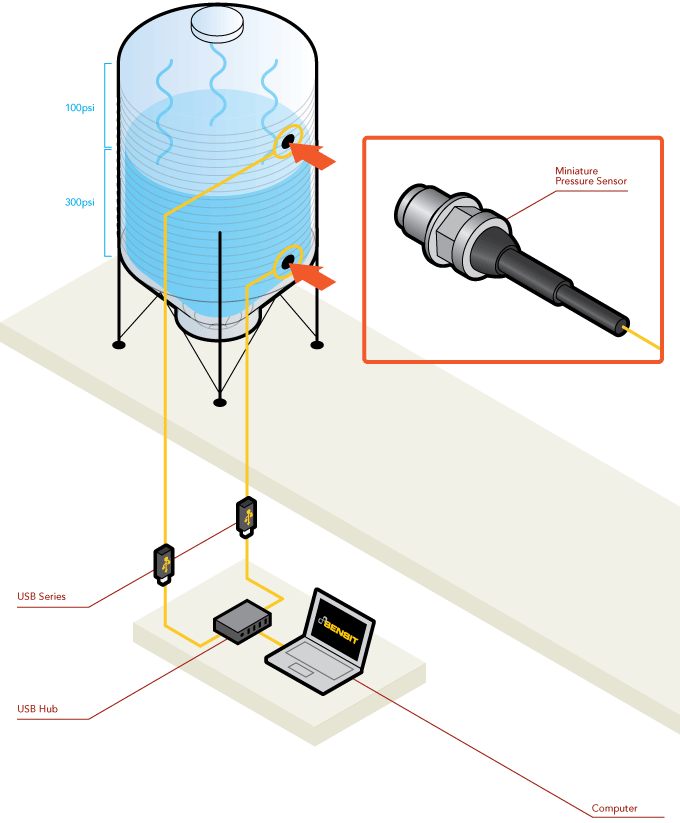Mode Layout
Theme color
Background Color:
Reset

# Differential Pressure Application

.

In some industrial applications, instead of a single pressure transducer that receives the pressure measurement from two different ports and compares it, two separate relative pressure transducers can be used. This may be the case when two different types of transducers are required due to media constraints (the difference in corrosion index of liquids requires a different transducer body), or even media differences (gas vs. liquid).

Using two differential pressure sensors and a control system to perform signal subtraction, process engineers can measure not only differential pressure but also level, flow, interface, and even density. The purpose of this article is not to cover all of these possibilities in detail, but rather to provide an overview of the range of secondary applications when using differential pressure sensors independently.

The bottom pressure measurement measures the total pressure exerted by the fluid and the gas above it, while the top pressure sensor measures only the static pressure exerted by the gases alone. This arrangement allows the gas pressure to be subtracted from the total bottom measurement, leaving the pressure generated by the liquid and allowing process engineers to determine the tank level, flow rate (rate of change of level) or indirectly the density of the liquid.

### h = (P2 - P1)/ (ρ * g)

h = height of the liquid column [m].

P2 = hydrostatic pressure at depth h

P1 = pressure of the gas enclosed in the tank

ρ = density of the liquid [kg/m³].

g = gravitational force [m/s²].

..

### How it works :

1. In this application, engineers and/or operators have installed two miniature pressure sensors to monitor the level of liquid and gas in the tank.
2. As shown, the fluid pressure in the tank measures 300 PSI, while the emitted gases measure 100 PSI.
3. These measurements are transmitted, via USB solutions, to a PC running SENSIT ™ test and measurement software. This software will calculate the differential pressure in the tank.
4. In this particular case, SENSIT ™ will calculate a differential pressure of 200 psi.